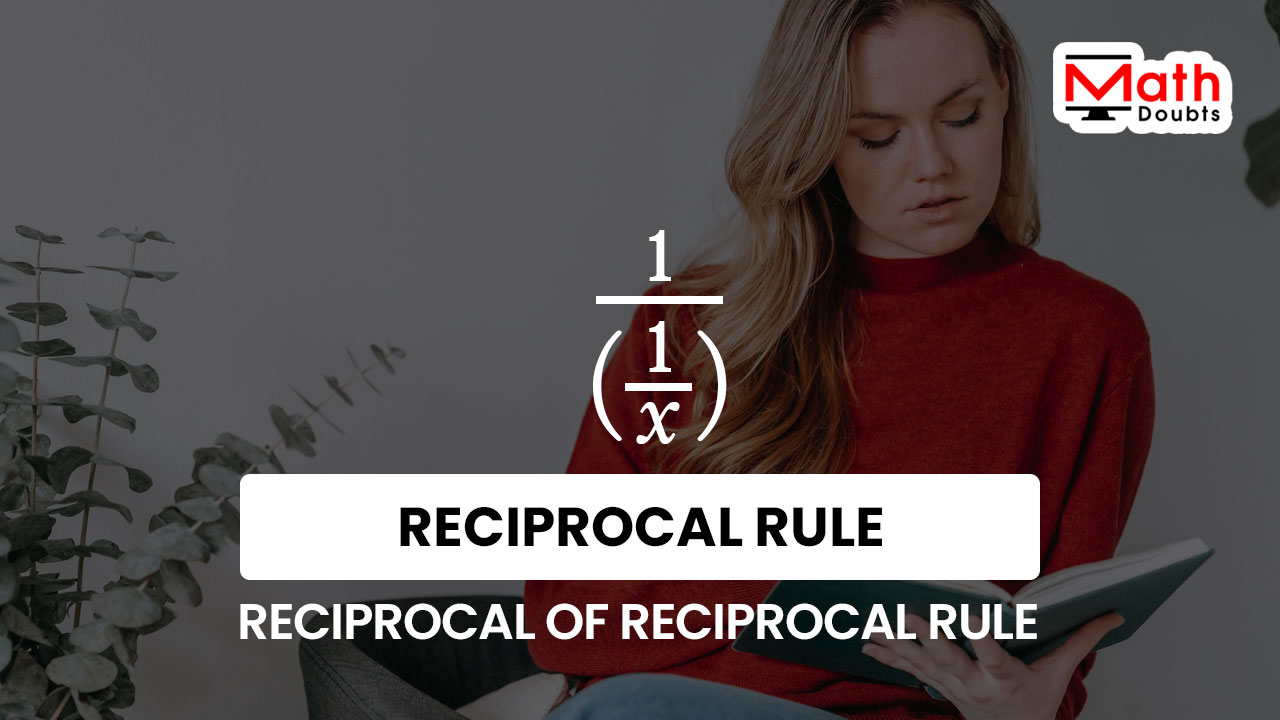# Reciprocal of a Reciprocal rule

## Formula

$\dfrac{1}{\bigg(\dfrac{1}{x}\bigg)} \,=\, x$

### Introduction

The reciprocal of a quantity is also appeared in reciprocal form in some cases in mathematics. The expression of such quantities confuses us in student level. So, a special formula is required to find the reciprocal of the reciprocal of quantities and it is called the reciprocal of a reciprocal rule.

It is time to learn what the reciprocal of a reciprocal rule is. Firstly, let’s denote a variable by a literal number $x$ and its reciprocal is written in mathematics as follows.

$\dfrac{1}{x}$

Now, the quotient of $1$ divided by $x$ is an algebraic function and it is called a reciprocal function. The reciprocal of the reciprocal function is mathematically expressed in the following form.

$\dfrac{1}{\bigg(\dfrac{1}{x}\bigg)}$

The reciprocal of the reciprocal of a variable $x$ is equal to the variable $x$ and it is called the reciprocal of a reciprocal rule.

$\,\,\,\therefore\,\,\,\,\,\,$ $\dfrac{1}{\bigg(\dfrac{1}{x}\bigg)} \,=\, x$#### Example

$\dfrac{1}{\bigg(\dfrac{1}{2}\bigg)} \,=\, 2$

##### Proof

Learn how to derive the reciprocal of a reciprocal rule to prove that the reciprocal of the reciprocal of a quantity is equal to the quantity.

Latest Math Topics
Jun 26, 2023
Jun 23, 2023

###### Math Questions

The math problems with solutions to learn how to solve a problem.

Learn solutions

Practice now

###### Math Videos

The math videos tutorials with visual graphics to learn every concept.

Watch now

###### Subscribe us

Get the latest math updates from the Math Doubts by subscribing us.